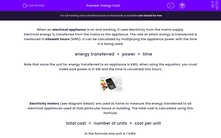# Use Kilowatt Hours and Calculate Energy Cost

In this worksheet, students will learn about domestic use of fuel and how the cost is calculated for bills.Key stage:  KS 3

Curriculum topic:   Physics: Energy

Curriculum subtopic:   Calculation of Fuel Uses and Costs

Difficulty level:#### Worksheet Overview

When an electrical appliance is on and working, it uses electricity from the mains supply. Electrical energy is transferred from the mains to the appliance. The rate at which energy is transferred is measured in kilowatt hours (kWh). It can be calculated by multiplying the appliance power with the time it is being used.

energy transferred  =  power  ×  time

Note that since the unit for energy transferred to an appliance is kWh, when using the equation, you must make sure power is in kW and the time is converted into hours.Electricity meters (see diagram below) are used at home to measure the energy transferred to all electrical appliances used at that particular house or building. The total cost is calculated using this formula:

total cost  =  number of units  ×  cost per unit

In the formula one unit is 1 kWh.It is essential that energy is used efficiently by everyone nowadays, as excessive use causes more pollution to the environment and costs more. All appliances waste some energy when on, so only a proportion of the energy is utilised for the actual intended use. For example, a light bulb uses electricity when lit, but some of the output energy is heat, which is not actually needed from a light bulb. Heat is wasted energy going into the environment. The more input energy (electricity) utilised by an appliance for the intended use, then the more efficient an appliance is.

Sankey diagrams show the input and output energy of appliances - you will see the Sankey diagram for a light bulb below. The electrical energy transferred to the light bulb is 100 J.  75 J are actually converted to light energy, which is the intended use of a light bulb.  25 J are wasted as heat.Let's put that information to use in this activity.

### What is EdPlace?

We're your National Curriculum aligned online education content provider helping each child succeed in English, maths and science from year 1 to GCSE. With an EdPlace account you’ll be able to track and measure progress, helping each child achieve their best. We build confidence and attainment by personalising each child’s learning at a level that suits them.

Get started